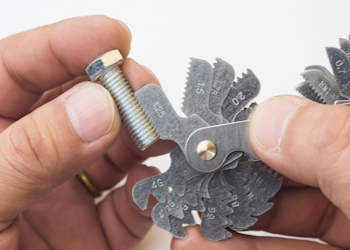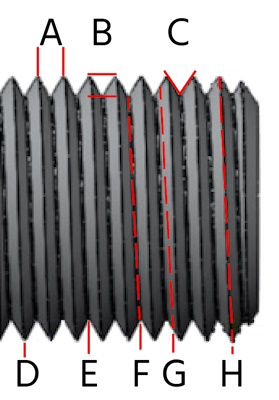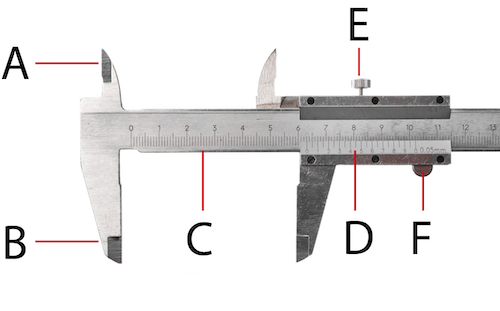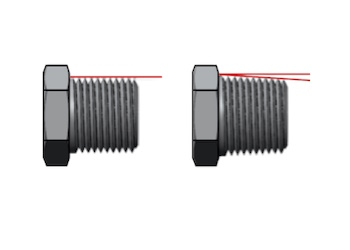# How To Measure Thread Size and PitchFigure 1: A pitch gauge to measure thread pitch

Measuring thread size, specifically the thread’s major diameter and pitch, is necessary to identify an unknown thread. The process is simple, using a caliper and a pitch gauge. This article describes using these tools and others, the methodology, and how to use the gathered data.

## Explore Tameson's selection of thread cutting toolsFigure 2: Thread dimensions: pitch (A), flank angle (B), minor diameter (C), pitch diameter (D), major diameter (E), depth (F), crest (G), and groove (H)

The following sections discuss measuring a threaded fastener's pitch, TPI, and major diameter.

• Pitch: Pitch (Figure 2 labeled A) is the distance between two consecutive peaks of a screw thread. It is measured in millimeters (mm) or inches (in).
• Threads-per-inch (TPI): Threads-per-inch (TPI) is a measure of the number of screw threads that are present on an inch of the screw's shaft. TPI is typically expressed in a number followed by a T, such as 16T, or 24T. A higher TPI indicates that there are more threads per inch, meaning the screw pitch is finer. For example, a screw with a TPI of 12 would have 12 threads per inch of total threading.
• Major diameter: The major diameter of a screw (Figure 2 labeled H) is the largest diameter of the screw thread. For external threads, it is measured from crest to crest. For internal threads, it is measured from root to root. typically used to identify a screw's size.

## How to measure threads on a screw

There are three thread measurement tools to determine the thread's major diameter and pitch- the Vernier caliper, a pitch gauge, and a ruler.

### Vernier caliper

A Vernier caliper (Figure 3) is the most helpful tool for measuring the major diameter of a threaded fastener, whether the threads are internal or external. The upper jaws on top of the caliper’s head (Figure 3 labeled A) can measure internal thread diameters, and the lower jaws (Figure 3 labeled B) can measure external thread diameters. The main scale (Figure 3 labeled C) shows the integer value of the measurement. This scale can be in centimeters or inches. The Vernier scale shows the decimal value of the measurement. On a metric scale, the Vernier scale represents 1 millimeter. The Vernier scale has 25 increments of 0.025 inches on an imperial scale.Figure 3: A close-up of a Vernier caliper scale with components: upper jaws (A), lower jaws (B), main scale (C), Vernier scale (D), lock screw (E), and thumb screw (F).

#### Measuring a thread with a Vernier caliper

When measuring the major diameter of a threaded fastener, first, it's essential to know if the thread is tapered. If a visual inspection cannot determine this, use the caliper to measure the fastener's first, fourth, and last threads. If the diameter changes across the fastener, the thread is tapered. If the diameter remains constant, the thread is straight or parallel (Figure 3).Figure 4: A straight male thread with a constant major diameter (left) and a tapered male thread with a varying major diameter (right)

1. Place an externally threaded bolt or screw between the lower jaws, or place the upper jaws inside an internally threaded fastener.
2. Use the thumb screw (Figure 3 labeled F) to bring the jaws into contact with the threading.
3. Upon contact, turn the lock screw (Figure 3 labeled E). The lock screw stops the caliper from adjusting while the measurement is being read.
4. Look at the 0 on the Vernier scale. The number on the main scale that the 0 matches determines the integer and first decimal of the measurement.
1. It is likely that 0 will not match perfectly with a number on the main scale but will rather be between two numbers.
5. Look at the division lines on the Vernier scale. The division line that lines up the closest with a line on the main scale determines the second decimal of the measurement.

The caliper in Figure 3 appears to open to the measurement of 6.31 cm. The 0 is at 6.3, and the line marked 1 on the Vernier scale matches up the closest with a line on the main scale.

#### Other notes

• Threads-per-inch: Position the caliper's jaws to span exactly one inch across the threaded portion to measure threads-per-inch. Count the number of complete thread peaks (or valleys) within that one-inch span with the caliper in place. This number is the TPI. Ensure to count the number of threads that are fully within the one-inch measurement. If a thread is only partially within this span, it should not be fully counted.

### Pitch measurement with a pitch gauge

Figure 1 shows a pitch gauge measuring a thread. Thread pitch gauges can be metric or imperial. A pitch gauge has several leaves with a number stamped on it. The number indicates the pitch. Having an imperial and metric gauge is important when identifying an unknown thread. There are similarities between metric and imperial threads that may lead to a false positive. For example, a metric pitch gauge may appear to match some imperial threads. An imperial gauge will have a closer match and provide the correct pitch.

### Measuring a thread with a ruler

A ruler can measure the major diameter and pitch of a threaded fastener. However, it's not as precise as using a caliper. The ruler should be high resolution and show measurements to a fraction of a millimeter. To measure the pitch of a thread in the United States or Canada, measure the threads-per-inch (TPI). To measure the pitch of a metric thread, measure the distance between two consecutive crests.

After measuring a thread’s major diameter and pitch, compare the results to thread standard charts to determine the thread’s standard. Thread standard charts have data for major diameter for external threads, minor diameter for internal threads, pitch, and tapping drill size. Get started by looking at our standard charts:

## FAQs

### How to measure thread size and pitch?

Use a high-precision ruler or a caliper to measure a thread's major diameter and pitch. For metric pitch, find the distance between two crests. For imperial pitch, find the threads-per-inch.